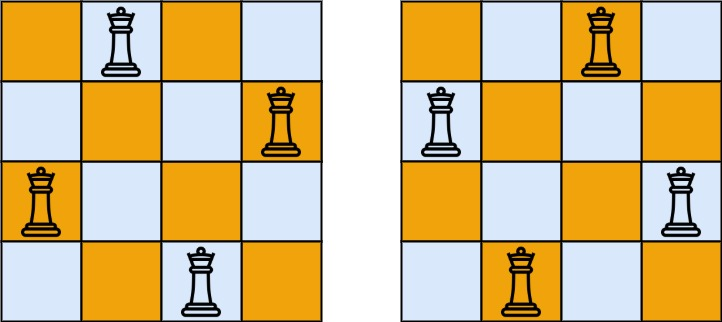# GeetCode Hub

The n-queens puzzle is the problem of placing `n` queens on an `n x n` chessboard such that no two queens attack each other.

Given an integer `n`, return all distinct solutions to the n-queens puzzle. You may return the answer in any order.

Each solution contains a distinct board configuration of the n-queens' placement, where `'Q'` and `'.'` both indicate a queen and an empty space, respectively.

Example 1:```Input: n = 4
Output: [[".Q..","...Q","Q...","..Q."],["..Q.","Q...","...Q",".Q.."]]
Explanation: There exist two distinct solutions to the 4-queens puzzle as shown above
```

Example 2:

```Input: n = 1
Output: [["Q"]]
```

Constraints:

• `1 <= n <= 9`

class Solution { public List<List<String>> solveNQueens(int n) { } }